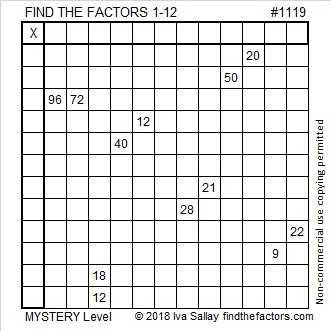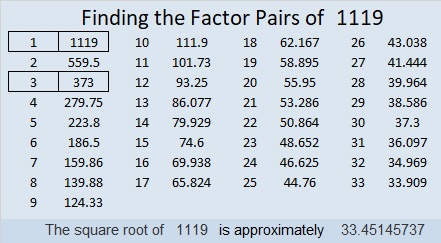# 1119 Mystery

Will this Mystery Level Puzzle be easy or difficult? That is part of the mystery! You might find the logic needed to solve it a mystery, too, but I assure you it can be solved without guessing and checking. Can you do it?Print the puzzles or type the solution in this excel file: 12 factors 1111-1119

Here are some facts about the number 1119:

• 1119 is a composite number.
• Prime factorization: 1119 = 3 × 373
• The exponents in the prime factorization are 1 and 1. Adding one to each and multiplying we get (1 + 1)(1 + 1) = 2 × 2 = 4. Therefore 1119 has exactly 4 factors.
• Factors of 1119: 1, 3, 373, 1119
• Factor pairs: 1119 = 1 × 1119 or 3 × 373
• 1119 has no square factors that allow its square root to be simplified. √1119 ≈ 33.451461119 is the sum of three consecutive prime numbers, one of which is its prime factor:
367 + 373 + 379 = 1119

1119 is the hypotenuse of a Pythagorean triple:
756-825-1119 which is 3 times (252-275-373)

1119 is a palindrome in two other bases:
It’s 383 in BASE 18 because 3(18²) + 8(18) + 3(1) = 1119, and
1H1 in BASE 26 (H is 17 base 10) because 1(26²) + 17(26) + 1(1) = 1119

This site uses Akismet to reduce spam. Learn how your comment data is processed.